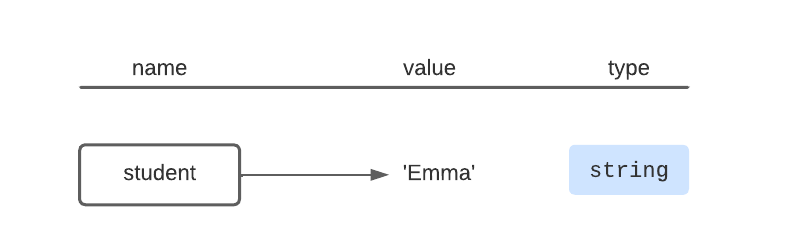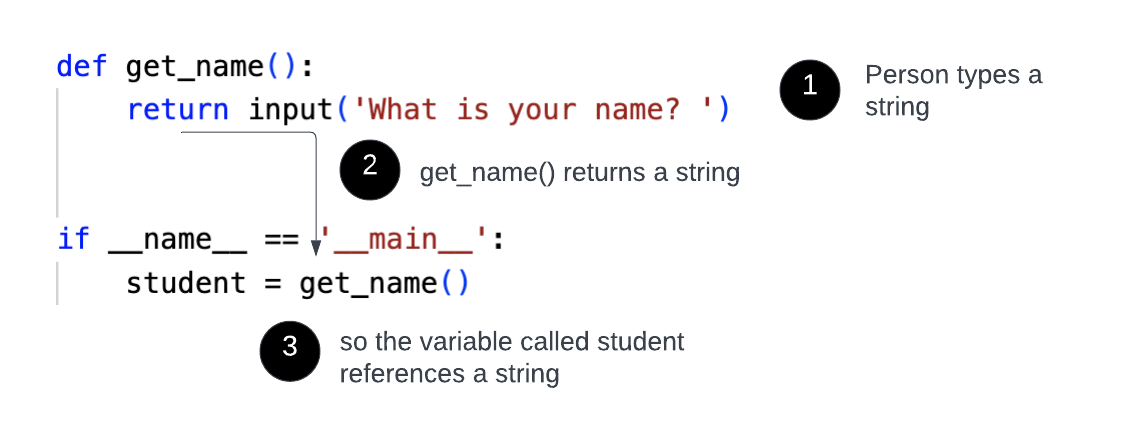Computer Science

# Types and operators

As you learn to program, it is helpful to understand some basic concepts that are common to all programming languages. Here we are going to cover types and operators.

## Types

Every value in Python has a type. We are going to cover three basic types: string, integer, and boolean.

### String

We have been using strings for some time, such as when we paint the color `'blue'`:

``bit.paint('blue')``

The type for the value `'blue'` is a string.

We have also seen strings when using `input()`:

``student = input('What is your name? ')``

If the person running your program types `Emma`, then the variable `student` references the string `'Emma'`.You can do likewise see this in the following code, where we directly set `student` equal to `'Emma'`:

``student = 'Emma'``

Remember that functions can return values. So in this code:

``````def get_name() -> str:
return input('What is your name? ')

if __name__ == '__main__':
student = get_name()``````

the variable `student` again has a type of string. This is because `get_name()` returns whatever `input()` returns, and `input()` returns a string:### Integer

Another type in Python is integer. You can see this below:

``number = 5``

Here, the variable `number` references the integer `5`.Note that there are no quotes around 5! If you instead type:

``number = '5'``

Then, the variable `number` now references the string `'5'`.

Just like with strings, functions can return integers:

``````def get_a_seven() -> int:
return 7

if __name__ = '__main__':
number = get_a_seven()``````

Here, `get_a_seven()` is a function that always returns 7, which is an integer. Likewise, the variable `number` eventually references the same number 7.

### Boolean

Finally, when you use `True` or `False`, then these have the boolean type. For example:

``having_a_great_time = True``

Here, the value `having_a_great_time` references the boolean `True`.Likewise, we can have a function that returns a boolean:

``````def i_am_hungry() -> bool:
return True

if __name__ == '__main__':
if i_am_hungry():

Here, the function `i_am_hungry()` returns `True`, which is a boolean, and because that boolean is True, the program will print `Now eating a salad`.

ValueType
‘hello’string
“why not?”string
3integer
Trueboolean
Falseboolean

## Operators

The type of a variable determines which operators you can use with it and what those operators do.

### Plus

For example, we can add integers with the `+` operator, and this works as you would expect:

``````if __name__ == '__main__':
print(10 + 7)``````

This will print `17`.

It may surprise you that in Python we can also use the `+` operator on strings:

``````if __name__ == '__main__':
print('fire' + 'place')``````

This will print `fireplace`, because for strings the `+` operator concatenates them.

### Minus

You can use the `-` operator to subtract integers:

``````if __name__ == '__main__':
print(10 - 7)``````

This will print `3`.

But you cannot use the `-` operator on strings! If you try it:

``````if __name__ == '__main__':
print('fire' - 'place')``````

you will see this error:

``````Traceback (most recent call last):
File "/Users/zappala/cs110/unit3/test.py", line 2, in <module>
print('fire' - 'place')
~~~~~~~^~~~~~~~~
TypeError: unsupported operand type(s) for -: 'str' and 'str'``````

This is telling you that `-` is an “unsupported operand” in between the types ‘str’ (string) and ‘str’ (string).

### Equality

Two check whether two values are equal, use two equal signs, `==`. Equality is a comparison operator, meaning you use it to compare two values:

``````if __name__ == '__main__':
number = 5
if number == 3:
elif number == 5:

Here, we first try `number == 3`, which is `False`. We next try `number == 5`, which is `True`. So this program will print: `Your number is 5`.

Try changing the first line of the main block to set `number` to a different value.

Similar to integers, we can use `==` to compare strings:

``````if __name__ == '__main__':
your_pet = 'cat'
if your_pet == 'dog':
print('A dog is a good second-best pet!')
elif your_pet == 'cat':
print('A cat is the best pet!')

``````

Here, we first try `your_pet == 'cat'`, which is `False`. We next try `your_pet == 'cat'`, which is `True`. So this program will print: `A cat is the best pet!`.

### Greater than and less than

Both `<` and `>` are also comparison operators.

We can use `>` to check if one value is greater than another:

``````if __name__ == '__main__':
number = 7
if number > 10:
elif number > 5:
else:

This will run the first comparison, `number > 10`, and it evaluates to `False`. The second comparison evaluates to `True`, so the program prints: `Your number is just right.`

We can likewise use `<` to compare values:

``````if __name__ == '__main__':
number = 7
if number < 5:
We can also use `>` and `<` to compare strings. This will do an alphabetical comparison for you. For example:
``````if __name__ == '__main__':
This will print `Animal 1 comes before animal 2`.